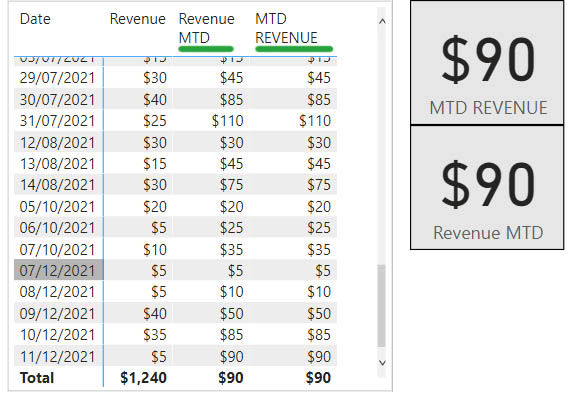top of page
Search
• Marek Vavrovic

# Time intelligence functions, part 04

## PREVIOUSDAY

Returns a previous day.

PREVIOUSDAY(<dates>)

Example

PREVIOUSDAY returns the day before the first day in the selection## PREVIOUSMONTH

Returns a previous month.

PREVIOUSMONTH(<dates>)

Example

Previous Month Rev =

CALCULATE([Revenue],PREVIOUSMONTH(DimCalendar[Date]))

Some months like Feb, Apr, Aug have no sales.## PREVIOUSYEAR

Returns a previous year.

PREVIOUSYEAR(<dates>[,<year_end_date>])

ExampleExample

In 2021 the company reached only 98.4% from the previous year sales volume. The revenue for 2021 was 1.6% lower than in the previous year.## STARTOFMONTH

Returns the start of month.

STARTOFMONTH(<dates>)

Example

STARTOFMONTH() vs ENDOFMONTH()

_START OF MONTH =

CALCULATE([Revenue MTD],STARTOFMONTH(DimCalendar[Date]))

_END OF MONTH =

CALCULATE([Revenue MTD],ENDOFMONTH(DimCalendar[Date]))

These functions return the beginning of the month or the end of the month, depends on the filter context. In this scenario \$15 is the first date of January 2020 and \$90 is the last date of December 2021.## STARTOFYEAR

Returns the start of year.

STARTOFYEAR(<dates>)

Example

STARTOFYEAR() vs ENDOFYEAR()

_START OF YEAR =

CALCULATE([Revenue MTD],STARTOFYEAR(DimCalendar[Date]))

_END OF YEAR =

CALCULATE([Revenue MTD],ENDOFYEAR(DimCalendar[Date]))

The value for _START OF YEAR measure is from 01.Jan.2020 and the value for _END OF YEAR measure is the last day of December 2020.## TOTALMTD

Evaluates the value of the expression for the month to date, in the current context.

Returns cumulative total in the month.

TOTALMTD(<expression>,<dates>[,<filter>])

This function is similar to DATESMTD()

Example

MTD REVENUE = TOTALMTD([Revenue],DimCalendar[Date])

Revenue MTD =

CALCULATE([Revenue],DATESMTD(DimCalendar[Date]))

Both functions return a running or cumulative total in the month. TOTALMTD() contains one extra optional argument [<filter>]## TOTALYTD

Evaluates the year-to-date value of the expression in the current context.

TOTALYTD(<expression>,<dates>[,<filter>][,<year_end_date>])

Function is similar to CALCULATE(<expression>, DATESYTD(<date>)), contains one extra optional argument [<filter>]

Example

REVENUE YTD =

CALCULATE([Revenue],DATESYTD(tblCustomers[Date]))

YTD REVENUE =

TOTALYTD([Revenue],tblCustomers[Date])

These functions calculate the running total in the year.Example

You can use the option [,<year_end_date>] argument to calculate the cumulative total for specified fiscal year.

FISCAL YEAR REVENUE =

TOTALYTD([Revenue],tblCustomers[Date],"2020-02-28")